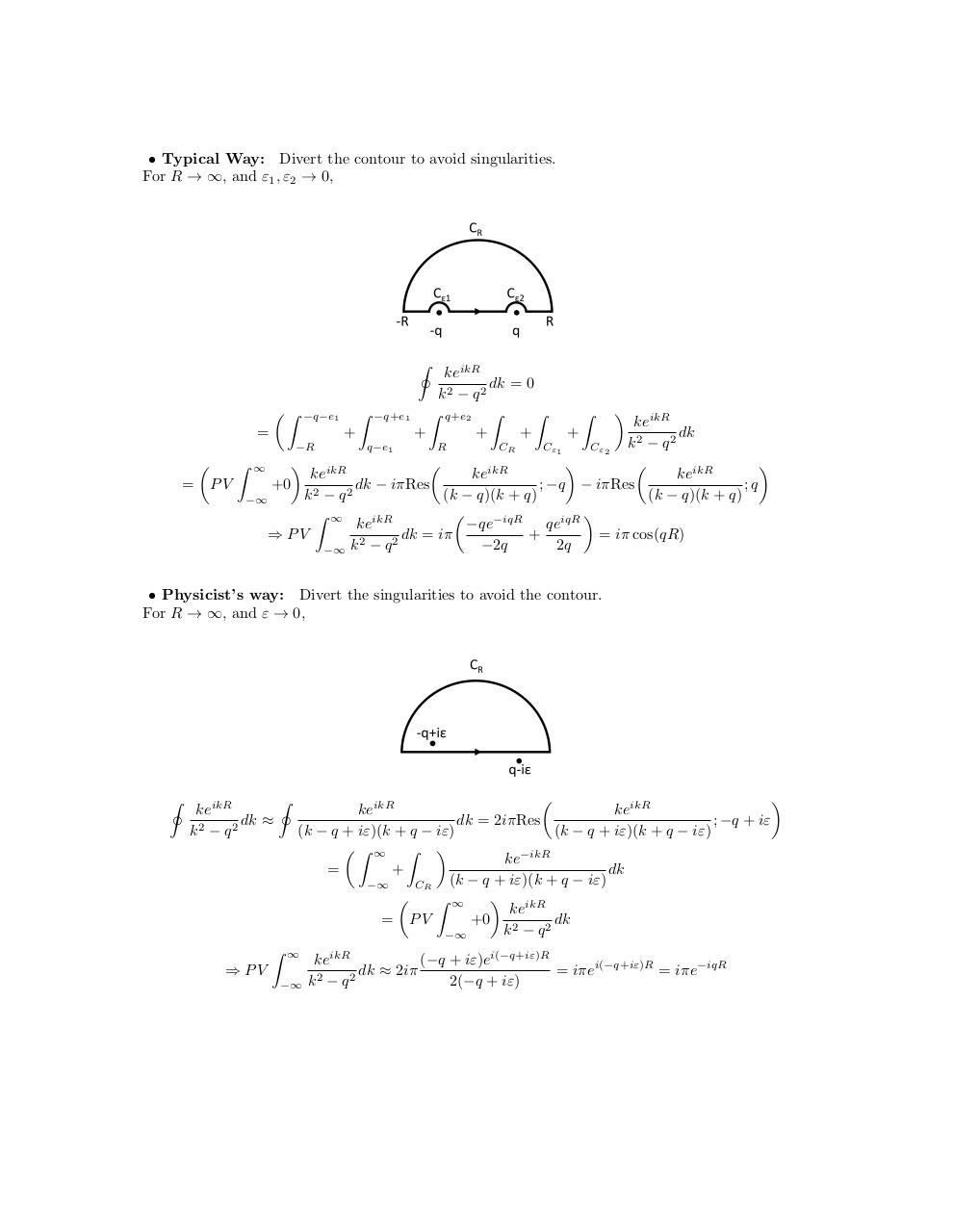# CauchyPV .pdf

### File information

Original filename: CauchyPV.pdf

This PDF 1.5 document has been generated by LaTeX with hyperref package / pdfTeX-1.40.16, and has been sent on pdf-archive.com on 28/06/2016 at 20:43, from IP address 173.250.x.x. The current document download page has been viewed 635 times.
File size: 149 KB (1 page).
Privacy: public file

CauchyPV.pdf (PDF, 149 KB)

### Document preview

• Typical Way: Divert the contour to avoid singularities.
For R → ∞, and ε1 , ε2 → 0,

keikR
dk = 0
k2 − q2
 Z −q−e1 Z −q+e1 Z q+e2 Z
Z
+
+
+
+
=
I

keikR
dk
k2 − q2
R
CR
Cε1
Cε2
−R
q−e1






Z ∞
keikR
keikR
keikR
dk

iπRes
;
−q

iπRes
;
q
= PV
+0 2
k − q2
(k − q)(k + q)
(k − q)(k + q)
−∞


Z ∞
qeiqR
keikR
−qe−iqR
⇒ PV
+
dk = iπ
= iπ cos(qR)
2
2
−2q
2q
−∞ k − q
Z



+

• Physicist’s way: Divert the singularities to avoid the contour.
For R → ∞, and ε → 0,

I

keikR
dk ≈
k2 − q2

I

Z



keikR
keikR
dk = 2iπRes
; −q + iε
(k − q + iε)(k + q − iε)
(k − q + iε)(k + q − iε)
Z ∞ Z 
−ikR
ke
dk
=
+
(k − q + iε)(k + q − iε)
−∞
CR


Z ∞
keikR
= PV
+0 2
dk
k − q2
−∞

⇒ PV
−∞

keikR
(−q + iε)ei(−q+iε)R
dk ≈ 2iπ
= iπei(−q+iε)R = iπe−iqR
2
2
k −q
2(−q + iε)#### HTML Code

Copy the following HTML code to share your document on a Website or Blog

#### QR Code### Related keywords